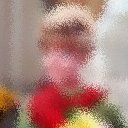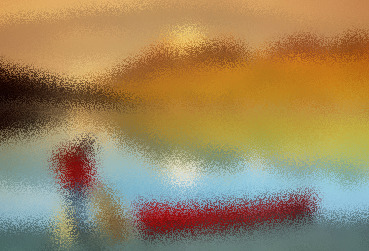## FROSTED

 Applies a frosted glass effect to an image.

 USAGE: frosted [-s spread] [-b bluramt] [-r reseed] infile outfile USAGE: frosted [-h or -help] -s .... spread ..... spread distance of dispersion; integer>=0; default=5 -b .... bluramt .... amount to blur image before dispersion; integer>=0; default=5 -r .... reseed ..... forced seed value; integer>0; default will randomly change seed PURPOSE: To apply a frosted glass effect to an image. DESCRIPTION: FROSTED applies a frosted glass effect to an image by using a sinusoidally modulaated random displacement map. ARGUMENTS: -s spread ... SPREAD distance of dispersion. Values are integers>=0. Typical values range from 2 to 20. The default=5 -b bluramt ... BLURAMT is the amount of blurring of the image in pixels before applying the dispersion. Values are integers>=0. The default=5. -r reseed ... RESEED is the forced seed value to use for randomization. This permits the pattern to be repeated. The default is to change the seed value randomly each time the script is run, thus causing somewhat different patterns each time the script is run. NOTE: For IM prior to 6.4.8-5, the script uses -fx and may be a little slow. CAVEAT: No guarantee that this script will work on all platforms, nor that trapping of inconsistent parameters is complete and foolproof. Use At Your Own Risk.

### EXAMPLES

 Example 1 Original Arguments: -b 3 -s 3 -r 100Original Arguments: -b 5 -s 5 -r 100What the script does is as follows: Blurs the image Creates a random displacement map Applies the displacement map to the image using a sinusoidal function This is equivalent to the following IM commands ww=`convert \$infile -format "%[fx:w]" info:` hh=`convert \$infile -format "%[fx:h]" info:` density=10 convert \$infile -blur 0x\${bluramt} \$infile` convert -size \${ww}x\${hh} xc: +noise Random -virtual-pixel tile \ -fx intensity -contrast-stretch 0% \$tmp0 convert \$infile \$tmp0 -monitor \ -fx "xx=i+\$spread*sin(\$density*v*2*pi); yy=j+\$spread*cos(\$density*v*2*pi); u.p{xx,yy}" \ \$outfile